14

用重抽样+主成分方法来做富集分析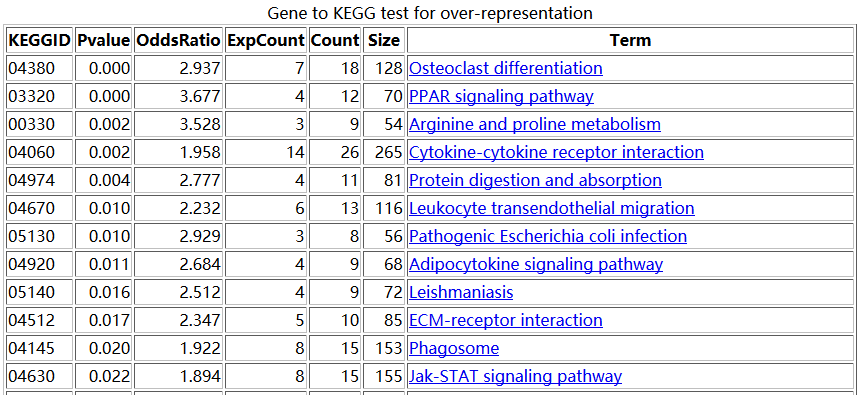setwd("D:\\my_tutorial\\补\\用limma包对芯片数据做差异分析")

rownames(exprSet)=exprSet[,1]

exprSet=exprSet[,-1]

exprSet=exprSet[,-c(1:2)]

library(hgu133plus2.db)

library(annotate)

platformDB="hgu133plus2.db";

probeset <- rownames(exprSet)

rowMeans <- rowMeans(exprSet)

EGID <- as.numeric(lookUp(probeset, platformDB, "ENTREZID"))

match_row=aggregate(rowMeans,by=list(EGID),max)

colnames(match_row)=c("EGID","rowMeans")

dat=data.frame(EGID,rowMeans,probeset)

tmp_prob=merge(dat,match_row,by=c("EGID","rowMeans"))

relevantProbesets=as.character(tmp_prob\$probeset)

length(relevantProbesets) #hgu133plus2.db  20156

exprSet=exprSet[relevantProbesets,]

EGID_name=as.numeric(lookUp(relevantProbesets, platformDB, "ENTREZID"))

rownames(exprSet)=as.character(EGID_name)

d=exprSet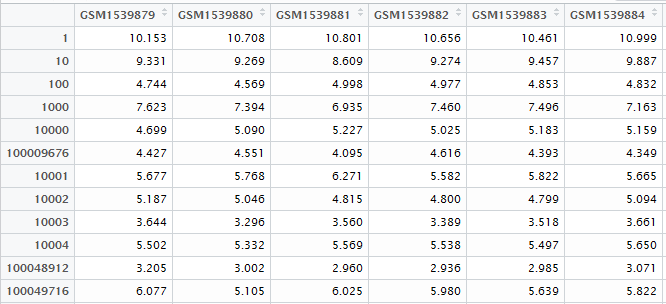gene128=sapply(1:1000,function(y) {

dat=t(d[sample(row.names(d), 128, replace=TRUE), ]);

round(100*summary(fast.prcomp(dat))\$importance[2,1],2)

}

)

>  summary(gene128)

Min. 1st Qu.  Median    Mean 3rd Qu.    Max.

19.1    25.8    28.8    29.8    32.5    59.7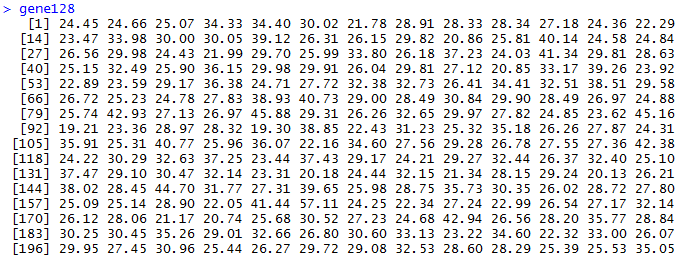path_04380_gene=intersect(rownames(d),as.character(Path2GeneID[['04380']]))

dat=t(d[path_04380_gene,]);

round(100*summary(fast.prcomp(dat))\$importance[2,1],2)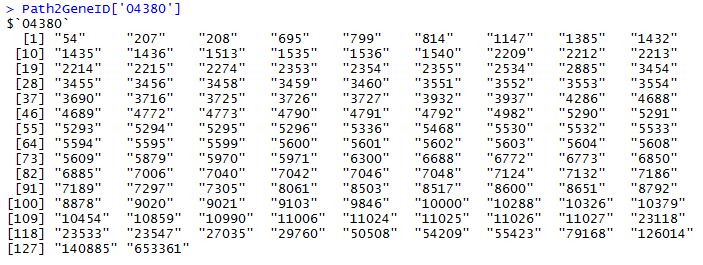1-pnorm((38.83-mean(gene128))/sd(gene128))

 0.0625

主成分分析略讲

options(digits = 2)
x=c(rnorm(5),rnorm(5)+4)
y=3*c(rnorm(5),rnorm(5)+4)
dat=rbind(x,y,a=0.1*x,b=0.2*x,c=0.3*x,o=0.1*y,p=0.2*y,q=0.3*y)
colnames(dat)=paste('s',1:10,sep="")
dat
library(gmodels)
pca=fast.prcomp(t(dat))
pca
summary(pca)\$importance
biplot(pca, cex=c(1.3, 1.2));

用超几何分布检验做富集分析

setwd("D:\\my_tutorial\\补\\用limma包对芯片数据做差异分析")

View(DEG)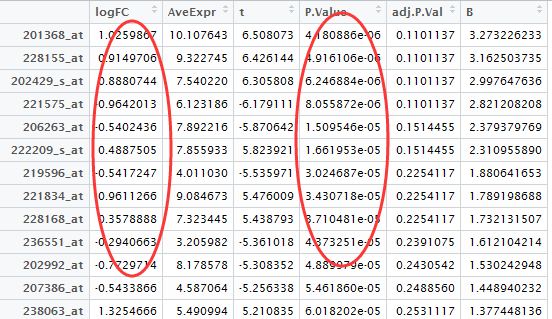probeset=rownames(DEG[abs(DEG[,1])>0.5 & DEG[,4]<0.05,])

library(hgu133plus2.db)

library(annotate)

platformDB="hgu133plus2.db";

EGID <- as.numeric(lookUp(probeset, platformDB, "ENTREZID"))

length(unique(EGID))

# 775

diff_gene_list <- unique(EGID)

library(GOstats)

library(org.Hs.eg.db)

#then do kegg pathway enrichment !

hyperG.params = new("KEGGHyperGParams", geneIds=diff_gene_list, universeGeneIds=NULL, annotation="org.Hs.eg.db",

categoryName="KEGG", pvalueCutoff=1, testDirection = "over")

KEGG.hyperG.results = hyperGTest(hyperG.params);tmp=toTable(org.Hs.egPATH)
GeneID2Path=tapply(tmp[,2],as.factor(tmp[,1]),function(x) x)
Path2GeneID=tapply(tmp[,1],as.factor(tmp[,2]),function(x) x)
#phyper(k-1,M, N-M, n, lower.tail=F)
#n*M/N
diff_gene_has_path=intersect(diff_gene_list,names(GeneID2Path))
n=length(diff_gene_has_path) #321 # 这里算出你总共抽取了多少个球
N=length(GeneID2Path) #5870  ##这里算出你总共有多少个球（这里是错的，有多少个球取决于背景基因！一般是两万个）
options(digits = 4)
for (i in names(Path2GeneID)){
M=length(Path2GeneID[[i]])  ##这个算出你的所有的球里面，白球有多少个
exp_count=n*M/N  ###这里算出你抽取的球里面应该多多少个是白色
k=0         ##这个k是你实际上抽取了多少个白球
for (j in diff_gene_has_path){
if (i %in% GeneID2Path[[j]]) k=k+1
}
OddsRatio=k/exp_count
p=phyper(k-1,M, N-M, n, lower.tail=F)  ##根据你实际上抽取的白球个数，就能算出富集概率啦！
print(paste(i,p,OddsRatio,exp_count,k,M,sep="    "))
}